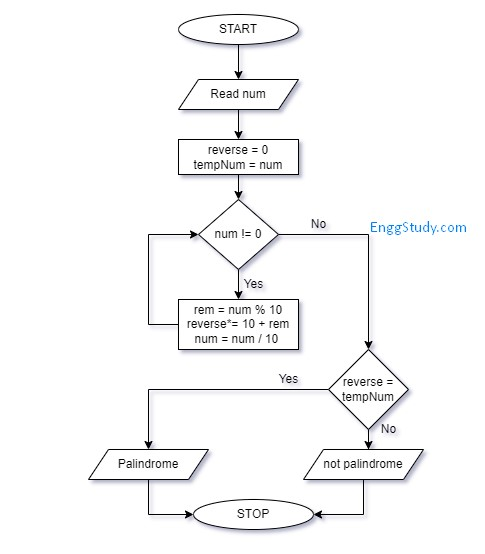# Check if number is Palindrome – Algorithm, Flowchart and Program

The palindrome number program is one of the most asked questions in the technical round during placement. Though the program is easy to write and understand many new students in the programming find it difficult to understand. In this article on the palindrome number, we will try to simplify the program and algorithm for you.

## What is a Palindrome or Palindrome number?

A palindrome number is a number that reads the same forwards and backwards. In simpler terms, if you have a number, and you read it from left to right or right to left, it remains the same.

For example, let’s suppose we have a number 121. If we read it from left to right, it is 121, and if we read it from right to left, it is still 121. In this case, we can say that the number 121 is a palindrome number.

Another example is the number 12321. If we read it from left to right, it is 12321, and if we read it from right to left, it is also 12321. So, the number 12321 is a palindrome number as well.

But, if we take the number 12345, and read it from left to right, it is 12345. However, if we read it from right to left, it becomes 54321. In this case, the number 12345 is not a palindrome number because it is different when read in reverse.

So, a palindrome number is a number that remains the same when read forwards or backwards.

for example,

121 <-> 121

3663 <-> 3663

Above are the palindrome number.

Now we are going to understand the palindrome number in the form of a flowchart so your concept about it will get clear.

## Flowchart to check if a given number is Palindrome or not## Algorithm to check if a given number is Palindrome or not

Step 1: Start

Step 2: Read the input number from the user

Step 3: Declare and initialize the variable reverse and assign input to a temp variable tempNum=num

Step 4: Start the while loop until num !=0 becomes false

• rem = num % 10
• reverse*= 10 + rem
• num = num / 10

Step 5 : Check if reverse == tempNum

Step 6: If it’s true then the number is a palindrome

Step 7: If not, the number is NOT a palindrome

Step 8: Stop

## Palindrome number in Java

Code for palindrome number in Java is given below:

``````import java.util.Scanner;

class Palindrome {

public static void main(String[] args){

Scanner sc = new Scanner(System.in);
System.out.println("Enter Number: ");
int num = sc.nextInt();

int reverse = 0;
int tempNum = num;

while(num>0){

int remainder = num % 10;

reverse = reverse*10 + remainder;

num = num / 10;

}

if(reverse == tempNum) {

System.out.println("Given number is Palindrome");
} else {
System.out.println("Given number is not Palindrome");
}

}

}``````

## Palindrome number in Python

Code for palindrome number in Python is given below:

``````num = int(input("Enter Number: "))

reverse = 0
tempNum = num

while num > 0:
remainder = num % 10
reverse = reverse * 10 + remainder
num = num // 10

if reverse == tempNum:
print("Given number is Palindrome")
else:
print("Given number is not Palindrome")``````

In this way, you can check if a given number is palindrome or not.

### 1 thought on “Check if number is Palindrome – Algorithm, Flowchart and Program”

1.Easy steps to learn